Two fifth-graders teams competing in math competitions - in Mathematical Olympiad and Pytagoriade. Of the 33 students competed in at least one of the contest 22 students. Students who competed only in Pytagoriade was twice more than those who just competed in the Mathematical Olympiad. Students who competed in both competitions was 4 times more than those who only competed in Pytagoriade.

a) How many students competed in both competitions?
b) How many students competed in just one competition?
c) How many students competed in no more than one competition?

Result

a =  16
b =  6
c =  17

Solution:

p+a+o = 22
p = 2 o
a = 4p
b = p+o
c = 33-a

a+o+p = 22
2o-p = 0
a-4p = 0
b-o-p = 0
a+c = 33

a = 16
b = 6
c = 17
o = 2
p = 4

Calculated by our linear equations calculator.

Leave us a comment of this math problem and its solution (i.e. if it is still somewhat unclear...):Be the first to comment!To solve this verbal math problem are needed these knowledge from mathematics:

Do you have a linear equation or system of equations and looking for its solution? Or do you have quadratic equation?

Next similar math problems:

1. Two numbersWe have two numbers. Their sum is 140. One-fifth of the first number is equal to half the second number. Determine those unknown numbers.
2. Families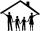In class we investigated how many children have family in which its live. How many% of pupils live in families with more than two children? If five families each have one child in 9 families have 2 children, in 5 families have 3 children, in 2 families ha
3. Find xSolve: if 2(x-1)=14, then x= (solve an equation with one unknown)
4. Three friendsThe three friends spent 600 KC in a teahouse. Thomas paid twice as much as Paul. Paul a half less than Zdeněk. How many each paid?
5. Three daysDuring the three days sold in stationery 1490 workbooks. The first day sold about workbooks more than third day. The second day 190 workbooks sold less than third day. How many workbooks sold during each day?
6. School year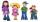At the end of the school year has awarded 20% of the 250 children who attend school. Awat got 18% boys and 23% of girls. Determine how many boys and how many girls attend school.
7. CandyPeter had a sachet of candy. He wanted to share with his friends. If he gave them 30 candies, he would have 62 candies. If he gave them 40 candies, he would miss 8 candies. How many friends did Peter have?
8. Borrowed book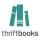Jane must as soon as possible return a borrowed book. She figured that when she read 15 pages a day return book in time. Then she read 18 pages a day and then return the book one day before. How many pages should have a book?
9. Parking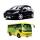120 vehicles parking on the morning. Pasenger car is charged 20 CZK, 50 CZK per bus. The guard collected for parking 2640 CZK in total. How many cars and how many buses stood in the parking?
10. Hotel roomsIn the 45 rooms, there were 169 guests, some rooms were three-bedrooms and some five-bedrooms. How many rooms were?
11. Belgium vs ItalyBelgium played a match with Italy and Belgium win by 2 goals. The match fell a total 6 goals. Determine the number of goals scored by Belgium and by Italy.
12. EquationSolve the equation: 1/2-2/8 = 1/10; Write the result as a decimal number.
13. Three workersThree workers were rewarded CZK 9200 and the money divided by the work they have done. First worker to get twice than the second, the second three times more than the third. How much money each worker received?
14. Tram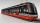In the three-part tram went 206 passengers in front of others, 226 after others and in the middle half of all. How many passengers went in tram total?
15. Spain vs USASpain lost to the US by 4 goals. In the match total fell 10 goals. How many goals gave the Spain and how the United States?
16. ClassroomThere are eighty more girls in the class than boys. Boys are 40 percent and girls are 60 percent. How many are boys and how many girls?
17. NormThree workers planted 3555 seedlings of tomatoes in one dey. First worked at the standard norm, the second planted 120 seedlings more and the third 135 seedlings more than the first worker. How many seedlings were standard norm?